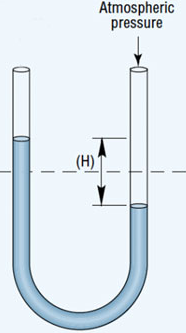# Problem: An open-end manometer containing mercury is connected to a container of gas, as depicted in Sample Exercise 10.2 in the textbook. What is the pressure of the enclosed gas in torr in each of the following situations?The mercury in the arm attached to the gas is 15.2 cm higher than in the one open to the atmosphere; atmospheric pressure is 0.985 atm .

###### FREE Expert Solution

The situation in the manometer described above should look like the image below where H= 15.2 cm:When the side exposed to the atmosphere (right) is lower than the side of the gas (left) we will use the equation below:

93% (180 ratings)###### Problem Details

An open-end manometer containing mercury is connected to a container of gas, as depicted in Sample Exercise 10.2 in the textbook. What is the pressure of the enclosed gas in torr in each of the following situations?

The mercury in the arm attached to the gas is 15.2 cm higher than in the one open to the atmosphere; atmospheric pressure is 0.985 atm .